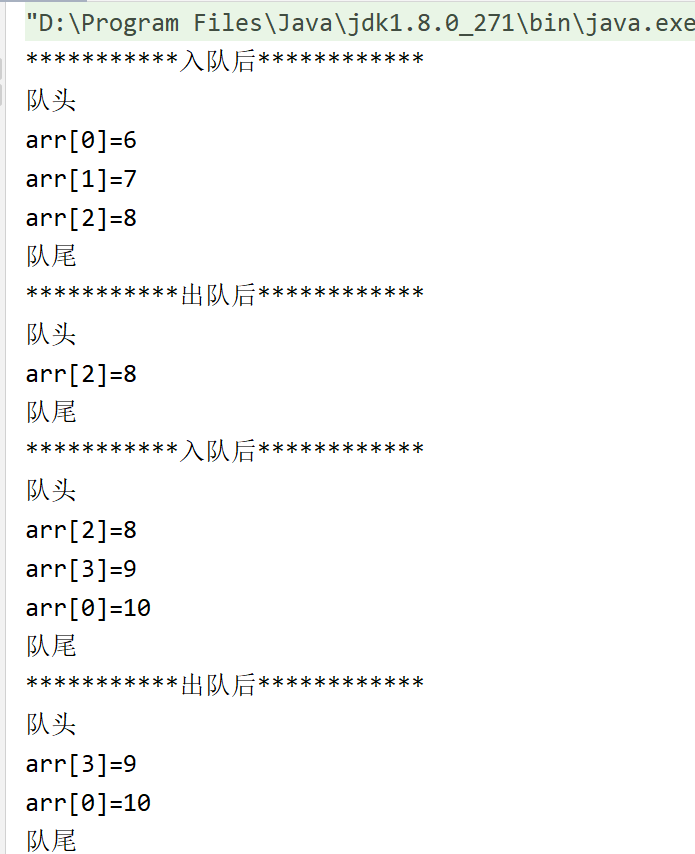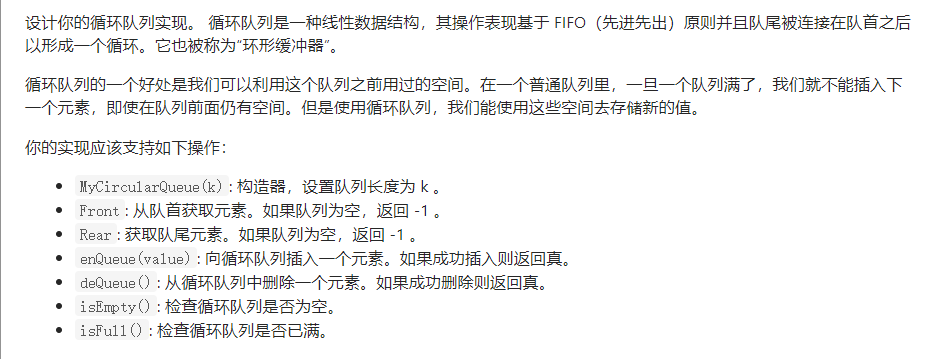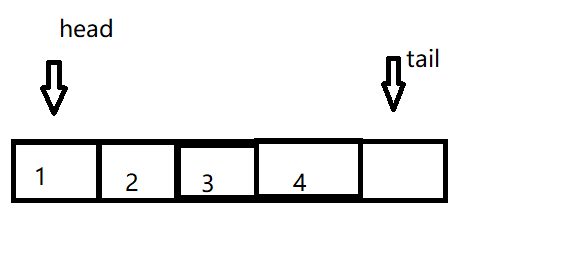• 学习笔记之数组实现循环队列——C语言 数组实现循环队列的逻辑性比指针实现循环链表更强，然而面试多用数组实现各个数据结构，需要把握好这类知识。 本次代码使用了数据域和尺寸来实现的。 判断循环队列空还是满都...

学习笔记之数组实现循环队列——C语言
数组实现循环队列的逻辑性比指针实现循环链表更强，然而面试多用数组来实现各个数据结构，需要把握好这类知识。
本次代码使用了数据域和尺寸来实现的。
判断循环队列空还是满都直接用到了Q->Size与0和Q->Capacity比较，更易看懂与接受。若不用数据域和指针，分为三种情况判断，具体如下。
1.可以让front指向队列的第一个元素，rear指向队列的最后一个元素的下一个位置；
2.也可以让front指向第一个位置的前一个位置，rear指向最后一个位置；
3.也可以让front指向第一个位置，rear指向最后一个位置
使用前两种方法可以使用front == rear - 1确定队列是否为空，第三种可以使用rear = front 来确定队列为空。
使用前两种方法可以使用front == rear确定队列是否为满，第三种可以使用rear = front - 1来确定队列为满。
使用第一种方法等于把从Q->Array开始存入数据，使用第二、三种方法等于从Q->Array开始存入数据。
本次代码使用第一种方法。

#include<stdio.h>
#include<stdlib.h>
#define Error( Str ) fprintf( stderr, "%s\n" , Str ),exit( 1 )
#define MinQueueSize 5

typedef int ElementType;

typedef struct Queue{
int Capacity;
int front;
int rear;
int Size;
ElementType *Array;
}*Queue;
//判断是否为空
int IsEmpty(Queue Q)
{
return Q->Size == 0;
}
//判断队列是否满
int IsFull(Queue Q)
{
if(Q->front != 0)
return Q->front == Q->rear;
else
return Q->Capacity == Q->Size;
}
//使队列为空
Queue MakeEmpty(Queue Q)
{
Q->front = 0;
Q->rear = 1;
Q->Size = 0;
return Q;
}
//创造一个队列
Queue CreatQueue(ElementType QueueSize)
{
Queue Q;

if(QueueSize <= MinQueueSize)
Error("Size too small");

Q = malloc(sizeof(struct Queue));
if(Q == NULL)
Error("Out of space!");

Q->Array = malloc(sizeof(ElementType) * QueueSize);
if(Q->Array == NULL)
Error("Out of space!!");

Q->Capacity = QueueSize;
MakeEmpty(Q);

return Q;
}
//入队
Queue EnQueue(Queue Q)
{
int Size;
ElementType X;
printf("请输入你要输入的队列的大小:");
scanf("%d",&Size);
if(IsFull(Q)||Size + Q->Size > Q->Capacity)
Error("Full Queue!");

Q->Size = Q->Size + Size;
for(;--Size != -1;)
{
printf("请输入你要输入的数据:");
scanf("%d",&X);
if(Q->rear == Q->Capacity)
Q->rear = 0;
Q->Array[Q->rear++] = X;
}
return Q;
}
//出队
Queue DeQueue(Queue Q)
{
if(IsEmpty(Q))
Error("Empty Queue");
if(++Q->front == Q->Capacity){
Q->front = 0;
}
Q->Size--;
return Q;
}
//不改变队列的情况输出队列
void printf_Queue(Queue Q)
{
int Size = Q->Size;
int Front = Q->front + 1;
for(;--Size != -1;){
if(Front == Q->Capacity)
Front = 0;
printf("%d ",Q->Array[Front++]);
}
}
//销毁队列
void DisposeQueue(Queue Q)
{
if(Q != NULL){
free(Q->Array);
free(Q);
}
}

int main()
{
Queue Q;
Q = CreatQueue(8);

Q = EnQueue(Q);
printf("队列为:");
printf_Queue(Q);

printf("\n");

Q = DeQueue(Q);
Q = DeQueue(Q);
Q = DeQueue(Q);
Q = DeQueue(Q);
Q = DeQueue(Q);
printf("五个数据出列后结果:");
printf_Queue(Q);

printf("\n");

Q = EnQueue(Q);
printf("队列为:");
printf_Queue(Q);

printf("\n");

Q = DeQueue(Q);
Q = DeQueue(Q);
Q = DeQueue(Q);
Q = DeQueue(Q);
Q = DeQueue(Q);

printf("五个数据出列后结果:");
printf_Queue(Q);

printf("\n");

DisposeQueue(Q);
}

展开全文c语言 数据结构
• 这里我用数组来模拟队列，并达到循环队列的效果 思路分析 首先定义一个数组，来存储数据。随后定义一个头指针front，指向队列的头元素；定义一个尾指针rear，指向待插入的位置，也就是队尾元素的后一个位置。 当头尾...

循环队列

队列是一种先进先出的顺序存储结构，尾端进，头端出。这里我使用数组来实现循环队列的效果。

思路分析

首先定义一个数组，来存储数据。随后定义一个头指针front，指向队列的头元素；定义一个尾指针rear，指向待插入的位置，也就是队尾元素的后一个位置。 当头尾指针指向同一个位置时，即rear == front的时候，队列为空，这个好理解。当 (rear+1)%capacity == front时，队列为满。具体请看图解。

图解线性看起来不直观，所以我又画了个环形的，请看下图。代码实现

public class Test {
private int[] arr;    // 数组实现循环队列
private int front;    // 队列的头指针
private int rear;     // 队列的尾指针
private int capacity; // 队列的最大容量

public Test(int capacity) {
this.capacity = capacity;
arr = new int[capacity];
}

public int deQueue() { //出队操作
int value = arr[front];
front = (front + 1) % capacity;
return value;
}

public void enQueue(int ele) { //入队操作
if (isFull()) {
System.out.println("队列满，不能添加");
return;
}
arr[rear] = ele;
rear = (rear + 1) % capacity;
}

public boolean isFull() { //判断是否为满
return (rear + 1) % capacity == front;
}

public boolean isEmpty() { //判断是否为空
return rear == front;
}

public String toString() { //重写toString()方法
if (isEmpty()) {
return "队列为空，没有数据！";
}
StringBuilder sb = new StringBuilder();
sb.append("队头\n");
for (int x = front; x < front + getSize(); x++) {
sb.append("arr[").append(x % capacity).append("]=").append(arr[x % capacity]).append("\n");
}
sb.append("队尾");
return sb.toString();
}

public int getSize() { //获取队列中实际存储的位置
return (rear - front + capacity) % capacity;
}

public static void main(String[] args) {
Test test = new Test(4);
test.enQueue(6); //入队
test.enQueue(7);
test.enQueue(8);
System.out.println("***********入队后************");
System.out.println(test);
test.deQueue();//出队
test.deQueue();
System.out.println("***********出队后************");
System.out.println(test);
System.out.println("***********入队后************");
test.enQueue(9);
test.enQueue(10);
System.out.println(test);
test.deQueue();
System.out.println("***********出队后************");
System.out.println(test);
}
}展开全文队列 数据结构 java 指针

循环队列优势在于仅在扩容时才进行数组复制操作，其余仅仅是根据tail索引添加元素，但会使用一个多余空间记录下一次添加时的索引，这样很好理解当first=tail时队列为空。
/**
* 数组实现循环队列普通方式
*
* 若使用first和last记录队首和队尾元素所在索引，当first=last时无法区分存在一个元素还是队列为空，需要增加size记录元素个数才能实现队列。
* 数组实现的普通队列添加一个元素后，first=0,下一个元素索引tail=1表明数组中存在一个元素，当first=0,tail=0时数组为空。
* 所以tail作为下一个元素的索引永远会占一个空间
* 循环队列同理，必须使用tail占用下一个元素的位置当first=tail时说明最后一个元素已经被取出或者未添加，则数组为空。
*
* @date: 2021/3/27 22:31
* @author: JiGang Q
*/
public class MyCircleQueue<T> implements MyQueue<T> {

private Object[] elements;
private static final int MIN_INITIAL_CAPACITY = 8;
private int first; //第一个元素
private int tail; //下次将要操作的索引，会在每次添加赋值后向“后”移动

public MyCircleQueue() {
this(MIN_INITIAL_CAPACITY + 1);
}

public MyCircleQueue(int size) {
if (size <= 0)
throw new IllegalArgumentException("size is not less than 0");
elements = new Object[size];
}

@Override
elements[tail] = t;
//末端扩容
if (tail + 1 == elements.length) {
if (first == 0) {
//正常扩容
Object[] newArr = new Object[elements.length<<1];
System.arraycopy(elements,0,newArr,0, elements.length);
elements = newArr;
tail++;
} else {
tail = 0;
}
}else if (tail + 1 == first) {
//中间扩容,还有种思路是放后面
Object[] newArr = new Object[elements.length<<1];
System.arraycopy(elements, first, newArr,0, elements.length - first);
System.arraycopy(elements,0,newArr,elements.length - first, first);
first = 0;
tail = elements.length;
elements = newArr;
} else {
tail++;
}
return true;
}

@Override
public T poll() {
if (first == tail)
return null;
T e = (T) elements[first];
elements[first] = null;
if (first == elements.length - 1)
first = 0;
else
first++;
return e;
}

@Override
public String toString() {
if (first == tail)
return "[]";
StringBuilder builder = new StringBuilder();
builder.append("[");
if (first < tail) {
for (int i = first; i < tail; i++)
builder.append(elements[i].toString() + ",");
} else {
for (int i = first; i < elements.length; i++)
builder.append(elements[i].toString() + ",");
for (int i = 0; i < tail; i++)
builder.append(elements[i].toString() + ",");
}
String str = builder.toString();
return str.substring(0,str.length() - 1) + "]";
}

}

使用求模实现循环队列

/**
*
* 使用求模实现队列
*
* @date: 2021/3/28 0:57
* @author: JiGang Q
*/
public class CircleQueue<T>  implements MyQueue<T>  {

private Object[] elements;
private static final int MIN_INITIAL_CAPACITY = 8;
private int first;
private int tail;

public CircleQueue() {
this(MIN_INITIAL_CAPACITY + 1);
}

public CircleQueue(int size) {
if (size <= 0)
throw new IllegalArgumentException("size is not less than 0");
elements = new Object[size];
}

@Override
elements[tail] = t;
grow();
tail = (tail+1)%elements.length;
return true;
}

//扩容
private void grow() {
if ((tail+1)%elements.length == first) {
Object[] newArr = new Object[elements.length<<1];
for (int i = 0; i < elements.length; i++)
newArr[i] = elements[(i + first)%elements.length];
tail = elements.length - 1;
elements = newArr;
first = 0;
}
}

@Override
public T poll() {
if (first == tail)
return null;
T e = (T) elements[first];
elements[first] = null;
first = (first + 1)%elements.length;
return e;
}

@Override
public String toString() {
if (first == tail)
return "[]";
StringBuilder builder = new StringBuilder();
builder.append("[");
for (int i = first; i != tail; i = (i + 1)%elements.length) {
builder.append(elements[i].toString() + ",");
}
String str = builder.toString();
return str.substring(0,str.length() - 1) + "]";
}

}

展开全文数据结构 队列 java
• 622. 设计循环队列 - 力扣（LeetCode） (leetcode-cn.com) 思路： ①根据给出的k值创建数组arr，大小为k 创建指针head表示头，tail表示尾，注意tail所指的下标不在队列之内 size用来表示当前队列中的有效...

622. 设计循环队列 - 力扣（LeetCode） (leetcode-cn.com)思路：

①根据给出的k值创建数组arr，大小为k

size用来表示当前队列中的有效元素的数量②要添加一个元素时

先判断队列是否为空，如果size=arr的长度了，队列已满，添加失败

如果小于，直接给tail所在的位置进行赋值，size++，同时tail移动到 下一个位置（tail+1）% arr.length

③要删除一个元素时

先判断队列内是否还有元素，如果size==0，说明没有元素，返回false；

⑤返回队尾元素，返回tail所在位置的上一个元素，即（tail-1+arr.length）%arr.length 所在位置的元素

class MyCircularQueue {
public int[]arr;
public int tail;
public int size;
public MyCircularQueue(int k) {
arr=new int[k];

}

public boolean enQueue(int value) {
if(size==arr.length){
return false;
}
arr[tail]=value;
tail=(tail+1)%(arr.length);
size++;
return true;
}

public boolean deQueue() {
if(size==0){
return false;
}
size--;
return true;
}

public int Front() {
if(size==0){
return -1;
}
}

public int Rear() {
if(size==0){
return -1;
}
int index=(tail-1+arr.length)%(arr.length);
return arr[index];
}

public boolean isEmpty() {
if(size==0){
return true;
}
return false;
}

public boolean isFull() {
if(size==arr.length){
return true;
}
return false;
}
}

展开全文java
• ﻿﻿/*** 数组实现循环队列**/public class LoopQueue{private Object[] elements;int capacity;int head;int rear;public LoopQueue(int capacity) {this.elements = new Object[capacity];this.capacity = ...
• class CircleArrayQueue {...//队列的长度private int[] queue; //队列private int head; //后指针private int tail; //前指针private static final int DEFALUT_SIZE = 10;public CircleArrayQueue() {this.size =...
• 队列是一种先进先出的的数据结构，我们同样可以使用数组、链表等来实现。我们可以在队列的尾部进行插入元素，在队列的头部取出元素。普通的队列由于空间利用率不高，所以我们一般都用循环队列循环队列中最重要的的...数组循环程序c语言
• 设计你的循环队列实现。...但是使用循环队列，我们能使用这些空间去存储新的值。 你的实现应该支持如下操作： MyCircularQueue(k): 构造器，设置队列长度为 k 。 Front: 从队首获取元素。如果队列为空，返回 -1 。
• 复习了下数据结构，Java的数组实现一下循环队列。队列的类//循环队列class CirQueue{private int QueueSize;private int front;private int rear;private int[] queueList ;public CirQueue(int QueueSize){this....
• 静态队列一般使用数组实现数组需要预先定义内存大小，为了避免内存浪费，一般使用循环队列。接下来讲述循环队列的原理以及实现代码。 循环队列数据结构定义： int front; //指向队列头，指向第一个数据节点 int ...c语言 队列 数据结构 指针
• 数组实现循环队列-Java package MyQueue; import java.lang.reflect.Array; public class MyQueueArray<AnyType> { private int theSize; private int currentSize; private Object[] theItems; private...队列 java
• 虽然实现了，但实际上并不需要每次都取余，...//循环队列 #define MAX 5 typedef struct Loop_que{ int base[MAX]; int rear; int size; int front; }loop; //初始化 loop* init(){ loop *l=(loop*)malloc(sizeof
• 下面小编就为大家带来一篇Java用数组实现循环队列的示例。小编觉得挺不错的，现在就分享给大家，也给大家做个参考。一起跟随小编过来看看吧复习了下数据结构，Java的数组实现一下循环队列。队列的类//循环队列...
• 数组实现循环队列（Java版） 什么是队列? 先进先出的线性数据结构 什么是循环队列？ ...本章循环队列使用数组取磨操作实现头尾下标位置变化。 取模操作：% 例如：5%3=2 3%6=3 循环队列各操作图数据结构 java 队列
• Java数组实现循环队列上一节(Java实现队列——顺序队列、链式队列)我们使用数组实现了顺序队列，但是在tail == n时会有数据搬移操作，这样入队操作性能就会受到影响。这里我们使用循环队列的解决思路。循环队列...
• 本篇文章小编给大家分享一下Java 1.8使用数组实现循环队列代码示例，文章代码介绍的很详细，小编觉得挺不错的，现在分享给大家供大家参考，有需要的小伙伴们可以来看看。1、引入使用数组实现循环队列，功能如下：1)...
• 我在上一篇博客《C语言实现使用静态数组实现循环队列》中实现使用静态数组来模拟队列的操作。由于数组的大小已经被指定，无法动态的扩展。所以在这篇博客中，我换成动态数组实现。动态数组可以不断开辟内存空间...c语言循环队列的数组
• 此方法实现循环队列的方式是在数组中空出来一位，从而达到循环的功能。 此空位的位置在添加删除数据时是不断变化的。 package CSDN; import java.util.Scanner; /**使用数组实现队列 * @author Babulakaka * @...
• 设计你的循环队列实现循环队列是一种线性数据结构，其操作表现基于 FIFO（先进先出）原则并且队尾被连接在队首之后以形成一个循环。它也被称为“环形缓冲器”。 循环队列的一个好处是我们可以利用这个队列之前...c语言 开发语言 数据结构...